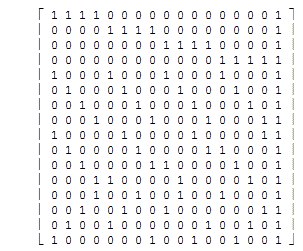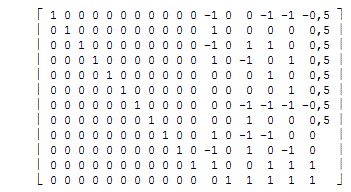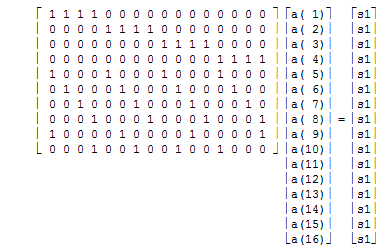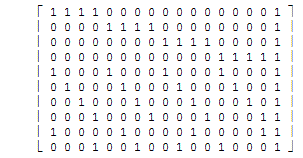Office Applications and Entertainment, Magic SquaresIndex About the Author

0.0 Introduction

A Magic Square of order n is an arrangement of n2 numbers, usually distinct integers, in a square, such that the n numbers in all rows, columns and diagonals sum to the same constant. A normal Magic Square contains the integers from 1 to n2.

Normal Magic Squares exist for all orders n >= 1 except n = 2, although the case n = 1 is trivial, consisting of a single cell containing the number 1. The smallest nontrivial case, shown below, is of order 3.

The constant sum in every row, column and diagonal is called the magic constant or magic sum. The magic constant of a normal Magic Square depends only on n and has the value s1 = n(n2 + 1)/2.

For normal Magic Squares of order n = 3, 4, 5, 6, ... the magic constants are: 15, 34, 65, 111, .....

Many papers about constructing Magic Squares have been published.

This page describes how Magic Squares can be constructed by means of elementary analytical methods used in solving couples of linear equations.

1.0 Magic Squares (3 x 3)

I got interested in Magic Squares in 1998, after I read a short article about the subject in NRC Handelsblad.

I wrote a short procedure in QuickBasic to generate possible Magic Squares by means of counting down, which worked quite well for Magic Squares of order 3, with only 9! = 362880 possibilities.

A translation of abovementioned procedure to VBA for Excel (MgcSqr3) still produces the same 8 possible solutions (in 2,23 seconds) for Magic Squares of order 3:

 2 7 6 9 5 1 4 3 8

 2 9 4 7 5 3 6 1 8
 4 3 8 9 5 1 2 7 6
 4 9 2 3 5 7 8 1 6
 6 1 8 7 5 3 2 9 4
 6 7 2 1 5 9 8 3 4
 8 1 6 3 5 7 4 9 2
 8 3 4 1 5 9 6 7 2

Note that each of the solutions above can be obtained from one of the others by rotation and/or reflection.

The method described above, failed however already for Magic Squares of order 4 with 16! = 2,1 1013 possibilities, which resulted in the development of the method described in following section(s).

2.0 Magic Squares (4 x 4)

2.1 Analytic Solution, Pan Magic Squares

A Magic Square for which all rows, columns, main - and broken diagonals sum to the same constant is normally referred to as a Pan Magic Square.

In general Magic Squares of order 4 can be represented as follows:

 a(1) a(2) a(3) a(4) a(5) a(6) a(7) a(8) a(9) a(10) a(11) a(12) a(13) a(14) a(15) a(16)

As the numbers a(i), i = 1 ... 16, in all rows, columns and diagonals sum to the same constant this results for Pan Magic Squares in following linear equations:

a( 1) + a( 2) + a( 3) + a( 4) = s1
a( 5) + a( 6) + a( 7) + a( 8) = s1
a( 9) + a(10) + a(11) + a(12) = s1
a(13) + a(14) + a(15) + a(16) = s1

a( 1) + a( 5) + a( 9) + a(13) = s1
a( 2) + a( 6) + a(10) + a(14) = s1
a( 3) + a( 7) + a(11) + a(15) = s1
a( 4) + a( 8) + a(12) + a(16) = s1

a( 1) + a( 6) + a(11) + a(16) = s1
a( 2) + a( 7) + a(12) + a(13) = s1
a( 3) + a( 8) + a( 9) + a(14) = s1
a( 4) + a( 5) + a(10) + a(15) = s1

a( 4) + a( 7) + a(10) + a(13) = s1
a( 3) + a( 6) + a( 9) + a(16) = s1
a( 2) + a( 5) + a(12) + a(15) = s1
a( 1) + a( 8) + a(11) + a(14) = s1

Or in matrix representation:Or shorter:

A * a = s1

The extended matrix of A can be written as:and reduced, by means of row and column manipulations, to:which results in the minimum number of linear equations:

a(13) =        s1 - a(14) - a(15) - a(16)
a(11) =        s1 - a(12) - a(15) - a(16)
a(10) =             a(12) - a(14) + a(16)
a(9)  =           - a(12) + a(14) + a(15)
a(8)  =  0.5 * s1 - a(14)
a(7)  = -0.5 * s1 + a(14) + a(15) + a(16)
a(6)  =  0.5 * s1 - a(16)
a(5)  =  0.5 * s1 - a(15)
a(4)  =  0.5 * s1 - a(12) + a(14) - a(16)
a(3)  =  0.5 * s1 + a(12) - a(14) - a(15)
a(2)  =  0.5 * s1 - a(12)
a(1)  = -0.5 * s1 + a(12) + a(15) + a(16)

Abovementioned reduction is achieved by means of a VBA for Excel procedure (Algorithm4), also a translation of a QuickBasic procedure, based on reduction algorithms as described in “Analytische Meetkunde II” by Dr. J. Bijl & Drs. W. J. H. Salet (1968).

The linear equations shown above, also generated by this procedure, are ready to be solved by the routine MgcSqr4 which will finally generate all possible Pan Magic Squares of order 4 for the defined magic constant (34).

The solutions are obtained by guessing a(12), a(14), a(15) and a(16) and filling out these guesses in the abovementioned equations which results in 384 Pan Magic Squares of the 4th order within 1,375 seconds.

The generated solutions of the 4th order Pan Magic Squares, for integers from 1 to 16 and related magic constant 34, are available in Attachment 1, showing all solutions, including those which can be obtained from one of the others by rotation or reflection.

2.2 Analytic Solution, Simple Magic Squares

If the requirements for Pan Magic Squares, as discussed in section 2.1 above, are moderated till the sum of all rows, columns and main diagonals sum to the same magic constant, the applicable equations are:

a( 1) + a( 2) + a( 3) + a( 4) = s1
a( 5) + a( 6) + a( 7) + a( 8) = s1
a( 9) + a(10) + a(11) + a(12) = s1
a(13) + a(14) + a(15) + a(16) = s1

a( 1) + a( 5) + a( 9) + a(13) = s1
a( 2) + a( 6) + a(10) + a(14) = s1
a( 3) + a( 7) + a(11) + a(15) = s1
a( 4) + a( 8) + a(12) + a(16) = s1

a( 1) + a( 6) + a(11) + a(16) = s1

a( 4) + a( 7) + a(10) + a(13) = s1

Or in matrix representation:Or shorter:

A * a = s1

The extended matrix of A can be written as:and reduced, by means of row and column manipulations, to:which results in the minimum number of linear equations:

a(13) =  s1 - a(14) - a(15) - a(16)
a( 9) =  s1 - a(10) - a(11) - a(12)
a( 7) =       a( 8) - a(10) + a(12) - a(13) + a(16)
a( 6) =  s1 - a( 8) - a(11) - a(12) + a(13) - a(16)
a( 5) =     - a( 8) + a(10) + a(11)
a( 4) =  s1 - a( 7) - a(10) - a(13)
a( 3) = -s1 - a( 8) + a( 9) + 2*a(10) + 2*a(13) +   a(14)
a( 2) =       a( 8) - a( 9) - 2*a(10) +   a(15) + 2*a(16)
a( 1) =       a( 8) + a(12) - a(13)

The linear equations shown above, are ready to be solved by the routine MgcSqr4b which will finally generate all possible Magic Squares of order 4 for the defined magic constant (34).

The solutions are obtained by guessing a(8), a(10), a(11), a(12), a(14), a(15) and a(16) and filling out these guesses in the abovementioned equations, which results in 7040 Magic Squares of the 4th order within 52,3 seconds.

The generated solutions of the 4th order Magic Squares, for integers from 1 to 16 and related magic constant 34, are available in Attachment 2.3.1.

The collection of 4th order Pan Magic Squares, as available in Attachment 1, is a sub collection of the Magic Squares shown in Attachment 2.3.1 and highlighted in red.

2.3 Analytic Solution, Associated Magic Squares

For Associated or Center Symmetric Magic Squares the sum of each pair of elements, which can be connected with a straight line through the center, equals to 2 * s1 / n = 17.

Consequently following equations should be added to the equations defining a Magic Square:

```a( 8) = 0.5 * s1 - a( 9)   a( 7) = 0.5 * s1 - a(10)
a( 6) = 0.5 * s1 - a(11)   a( 5) = 0.5 * s1 - a(12)
a( 4) = 0.5 * s1 - a(13)   a( 3) = 0.5 * s1 - a(14)
a( 2) = 0.5 * s1 - a(15)   a( 1) = 0.5 * s1 - a(16)
```

which results in following linear equations describing Associated Magic Squares:

```a(13) =       s1 - a(14) - a(15) - a(16)
a(11) =       s1 - a(12) - a(15) - a(16)
a(10) =       s1 - a(12) - a(14) - a(16)
a( 9) =       s1 - a(10) - a(11) - a(12)
a( 8) = 0.5 * s1 - a( 9)
a( 7) = 0.5 * s1 - a(10)
a( 6) = 0.5 * s1 - a(11)
a( 5) = 0.5 * s1 - a(12)
a( 4) = 0.5 * s1 - a(13)
a( 3) = 0.5 * s1 - a(14)
a( 2) = 0.5 * s1 - a(15)
a( 1) = 0.5 * s1 - a(16)
```

The solutions can be obtained by guessing a(16), a(15), a(14) and a(12) and filling out these guesses in the equations shown above.

An optimized guessing routine (MgcSqr4b2), produced 384 Associated Magic Squares within 8,7 seconds, which are shown in Attachment 2.5.

2.4 Analytic Solution, Most Perfect Magic Square

A Most Perfect Magic Square of order n is defined as a Magic Square containing the numbers 1 to n2 with two additional properties:

1. Compact : Each 2 × 2 sub square sums to 2 * (n2 + 1);
2. Complete: All pairs of integers distant n/2 along a (main) diagonal sum to (n2 + 1).

To determine all Most Perfect Magic Squares of order 4 the following equations should be added to the equations listed in section 2.2 above:

 a( 1) + a( 2) + a( 5) + a( 6) = s1 a( 2) + a( 3) + a( 6) + a( 7) = s1 a( 3) + a( 4) + a( 7) + a( 8) = s1 a( 1) + a( 4) + a( 5) + a( 8) = s1 a( 5) + a( 6) + a( 9) + a(10) = s1 a( 6) + a( 7) + a(10) + a(11) = s1 a( 7) + a( 8) + a(11) + a(12) = s1 a( 5) + a( 8) + a( 9) + a(12) = s1 a( 9) + a(10) + a(13) + a(14) = s1 a(10) + a(11) + a(14) + a(15) = s1 a(11) + a(12) + a(15) + a(16) = s1 a( 9) + a(12) + a(13) + a(16) = s1 a(13) + a(14) + a( 1) + a( 2) = s1 a(14) + a(15) + a( 2) + a( 3) = s1 a(15) + a(16) + a( 3) + a( 4) = s1 a(13) + a(16) + a( 1) + a( 4) = s1 a( 1) + a(11) = s1/2 a( 4) + a(10) = s1/2 a( 6) + a(16) = s1/2 a( 7) + a(13) = s1/2

The resulting number of equations can be written in the matrix representation as:

A * a = s

which can be reduced, by means of row and column manipulations, and results in following set of linear equations:

a(13) =        s1 - a(14) - a(15) - a(16)
a(11) =        s1 - a(12) - a(15) - a(16)
a(10) =             a(12) - a(14) + a(16)
a(9)  =           - a(12) + a(14) + a(15)
a(8)  =  0.5 * s1 - a(14)
a(7)  = -0.5 * s1 + a(14) + a(15) + a(16)
a(6)  =  0.5 * s1 - a(16)
a(5)  =  0.5 * s1 - a(15)
a(4)  =  0.5 * s1 - a(12) + a(14) - a(16)
a(3)  =  0.5 * s1 + a(12) - a(14) - a(15)
a(2)  =  0.5 * s1 - a(12)
a(1)  = -0.5 * s1 + a(12) + a(15) + a(16)

The linear equations shown above are identical to the linear equations found in section 2.1 for Pan Magic Squares of the 4th order, so all Pan Magic Squares of order 4, as shown in Attachment 1, are Most Perfect Magic Squares.

As a consequence of the defining properties listed above, following additional properties can be recognized for Pan Magic Squares:

1. The corners of each 3 x 3 and 4 x 4 square sum to the magic constant;
2. The two sets of four symmetrical numbers, a(3)+a(8)+a(9)+a(14) and a(2)+a(5)+a(12)+a(15), sum to the magic constant;
3. The middle two numbers of the two outer columns sum to the magic constant;
4. The middle two numbers of the two outer rows sum to the magic constant;
5. Eight trapeziums can be recognized, which sum to the magic constant;
6. Four "triangles" can be recognized, which sum to the magic constant.

Which is illustrated below for the Pan Magic Square as shown in the spreadsheet solution CnstrSngl4c.

Corners 3 x 3
 7 12 1 14 2 13 8 11 16 3 10 5 9 6 15 4

 7 12 1 14 2 13 8 11 16 3 10 5 9 6 15 4

 7 12 1 14 2 13 8 11 16 3 10 5 9 6 15 4

 7 12 1 14 2 13 8 11 16 3 10 5 9 6 15 4
Corners 4 x 4
 7 12 1 14 2 13 8 11 16 3 10 5 9 6 15 4

Symetrical Pairs
 7 12 1 14 2 13 8 11 16 3 10 5 9 6 15 4

 7 12 1 14 2 13 8 11 16 3 10 5 9 6 15 4
Outer Clmns
 7 12 1 14 2 13 8 11 16 3 10 5 9 6 15 4
Outer Rows
 7 12 1 14 2 13 8 11 16 3 10 5 9 6 15 4
Trapeziums
 7 12 1 14 2 13 8 11 16 3 10 5 9 6 15 4

 7 12 1 14 2 13 8 11 16 3 10 5 9 6 15 4

 7 12 1 14 2 13 8 11 16 3 10 5 9 6 15 4

 7 12 1 14 2 13 8 11 16 3 10 5 9 6 15 4

 7 12 1 14 2 13 8 11 16 3 10 5 9 6 15 4

 7 12 1 14 2 13 8 11 16 3 10 5 9 6 15 4

 7 12 1 14 2 13 8 11 16 3 10 5 9 6 15 4

 7 12 1 14 2 13 8 11 16 3 10 5 9 6 15 4
"Triangles"
 7 12 1 14 2 13 8 11 16 3 10 5 9 6 15 4

 7 12 1 14 2 13 8 11 16 3 10 5 9 6 15 4

 7 12 1 14 2 13 8 11 16 3 10 5 9 6 15 4

 7 12 1 14 2 13 8 11 16 3 10 5 9 6 15 4

All these properties, together with the defining properties, can be tested in a spread sheet solution (ref. CnstrSngl4c).

2.5 Other Magic Constants

Other magic constants are possible for both Magic Squares and Pan Magic Squares.

With procedure MgcSqr4c, 3213696 Pan Magic Squares of the 4th order with magic constant 130 can be generated, based on the equations developed in section 2.1 above for distinct integers from 1 to 64.

The number of Pan Magic Squares of the 4th order with s1 = 130 are broken down below for i = a(16) = 1, 2, ... 64:

 i n(i) i n(i) i n(i) i n(i) i n(i) i n(i) i n(i) i n(i) 1 27576 9 43896 17 54288 25 59904 33 58440 41 59280 49 53688 57 42144 2 29784 10 45816 18 55224 26 59688 34 59424 42 58920 50 52152 58 40656 3 32088 11 47064 19 56424 27 59640 35 59088 43 58896 51 51096 59 38424 4 34560 12 48504 20 56688 28 60072 36 59376 44 57408 52 50256 60 36384 5 36384 13 50256 21 57408 29 59376 37 60072 45 56688 53 48504 61 34560 6 38424 14 51096 22 58896 30 59088 38 59640 46 56424 54 47064 62 32088 7 40656 15 52152 23 58920 31 59424 39 59688 47 55224 55 45816 63 29784 8 42144 16 53688 24 59280 32 58440 40 59904 48 54288 56 43896 64 27576

In section 8.2 will be discussed how 8th order Magic Squares and Pan Magic Squares can be constructed out of 4th order (Pan) Magic Squares.